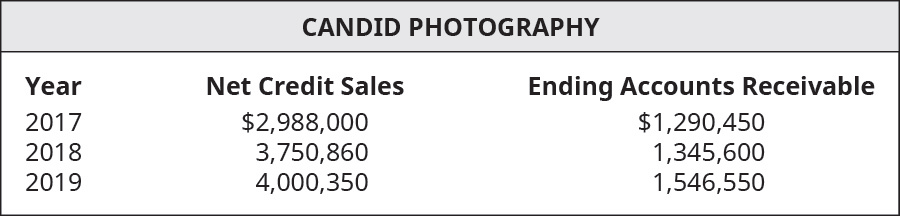# The following select financial statement information from Candid Photography. Compute the accounts receivable turnover ratios and the number of days’ sales in receivables ratios for 2018 and 2019 (round answers to two decimal places). What do the outcomes tell a potential investor about Candid Photography if industry average for accounts receivable turnover ratio is 3 times and days’ sales in receivables ratio is 150 days?FindFindarrow_forward

### Principles of Accounting Volume 1

19th Edition
OpenStax
Publisher: OpenStax College
ISBN: 9781947172685

#### Solutions

Chapter
SectionFindFindarrow_forward

### Principles of Accounting Volume 1

19th Edition
OpenStax
Publisher: OpenStax College
ISBN: 9781947172685
Chapter 9, Problem 8PA
Textbook Problem
1 views

## The following select financial statement information from Candid Photography.Compute the accounts receivable turnover ratios and the number of days’ sales in receivables ratios for 2018 and 2019 (round answers to two decimal places). What do the outcomes tell a potential investor about Candid Photography if industry average for accounts receivable turnover ratio is 3 times and days’ sales in receivables ratio is 150 days?

To determine

Accounts receivable turnover ratio:

This is an efficiency ratio that indicates the conversion of accounts receivable into cash. This ratio is calculated by dividing the Net credit Sales by the Average accounts receivable. The formula to calculate this ratio is as follows:

Accounts receivable turnover ratio = Net credit SalesAverage accounts receivable

Days Sales uncollected:

This isan efficiency ratio that indicates the period for which credit sales remain as receivable. The ratio is calculated by multiplying Accounts receivable with 365 days and dividing the result by Net Sales. The formula for Days Sales uncollected is as follows:

Days Sales uncollected = Accounts Receivable * 365Net Sales

To calculate:

The Accounts receivable turnover ratio and Days sales in receivable for each year and indications to potential investors.

### Explanation of Solution

The Accounts receivable turnover ratio and Days sales in receivable for each year are calculated as follows:

...
 Year 2018 Year 2019 Net Credit Sales (A) $3,750,860$ 4,000,350 Beginning Accounts Receivable (B) $1,290,450$ 1,345,600 Ending Accounts Receivable (C) $1,345,600$ 1,546,550 Average Accounts Receivables (D) = (B+C)÷2

### Still sussing out bartleby?

Check out a sample textbook solution.

See a sample solution

#### The Solution to Your Study Problems

Bartleby provides explanations to thousands of textbook problems written by our experts, many with advanced degrees!

Get Started

Find more solutions based on key concepts
Explain how a company can report a loss and still have a positive net operating cash flow.

Managerial Accounting: The Cornerstone of Business Decision-Making

As a first step, we need to estimate what percentage of MMMs capital comes from debt, preferred stock, and comm...

Fundamentals of Financial Management, Concise Edition (with Thomson ONE - Business School Edition, 1 term (6 months) Printed Access Card) (MindTap Course List)

How are inflation and unemployment related in the short run?

Principles of Economics (MindTap Course List)

Define the term product

MKTG 12:STUDENT ED.-TEXT

What rights do stockholders have?

Foundations of Business (MindTap Course List)